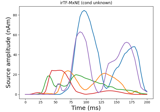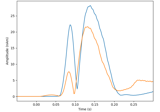# mne.inverse_sparse.tf_mixed_norm#

mne.inverse_sparse.tf_mixed_norm(evoked, forward, noise_cov, loose='auto', depth=0.8, maxit=3000, tol=0.0001, weights=None, weights_min=0.0, pca=True, debias=True, wsize=64, tstep=4, window=0.02, return_residual=False, return_as_dipoles=False, alpha=None, l1_ratio=None, dgap_freq=10, rank=None, pick_ori=None, n_tfmxne_iter=1, verbose=None)[source]#

Time-Frequency Mixed-norm estimate (TF-MxNE).

Compute L1/L2 + L1 mixed-norm solution on time-frequency dictionary. Works with evoked data 12.

Parameters
evokedinstance of `Evoked`

Evoked data to invert.

forward`dict`

Forward operator.

noise_covinstance of `Covariance`

Noise covariance to compute whitener.

loose`float` | ‘auto’ | `dict`

Value that weights the source variances of the dipole components that are parallel (tangential) to the cortical surface. Can be:

• float between 0 and 1 (inclusive)

If 0, then the solution is computed with fixed orientation. If 1, it corresponds to free orientations.

• `'auto'` (default)

Uses 0.2 for surface source spaces (unless `fixed` is True) and 1.0 for other source spaces (volume or mixed).

• dict

Mapping from the key for a given source space type (surface, volume, discrete) to the loose value. Useful mostly for mixed source spaces.

depth

How to weight (or normalize) the forward using a depth prior. If float (default 0.8), it acts as the depth weighting exponent (`exp`) to use None is equivalent to 0, meaning no depth weighting is performed. It can also be a `dict` containing keyword arguments to pass to `mne.forward.compute_depth_prior()` (see docstring for details and defaults). This is effectively ignored when `method='eLORETA'`.

Changed in version 0.20: Depth bias ignored for `method='eLORETA'`.

maxit`int`

Maximum number of iterations.

tol`float`

Tolerance parameter.

weights

Weight for penalty in mixed_norm. Can be None or 1d array of length n_sources or a SourceEstimate e.g. obtained with wMNE or dSPM or fMRI.

weights_min`float`

Do not consider in the estimation sources for which weights is less than weights_min.

pcabool

If True the rank of the data is reduced to true dimension.

debiasbool

Remove coefficient amplitude bias due to L1 penalty.

wsize

Length of the STFT window in samples (must be a multiple of 4). If an array is passed, multiple TF dictionaries are used (each having its own wsize and tstep) and each entry of wsize must be a multiple of 4. See 3.

tstep

Step between successive windows in samples (must be a multiple of 2, a divider of wsize and smaller than wsize/2) (default: wsize/2). If an array is passed, multiple TF dictionaries are used (each having its own wsize and tstep), and each entry of tstep must be a multiple of 2 and divide the corresponding entry of wsize. See 3.

window

Length of time window used to take care of edge artifacts in seconds. It can be one float or float if the values are different for left and right window length.

return_residualbool

If True, the residual is returned as an Evoked instance.

return_as_dipolesbool

If True, the sources are returned as a list of Dipole instances.

alpha`float` in [0, 100) or `None`

Overall regularization parameter. If alpha and l1_ratio are not None, alpha_space and alpha_time are overridden by alpha * alpha_max * (1. - l1_ratio) and alpha * alpha_max * l1_ratio. 0 means no regularization, 100 would give 0 active dipole.

l1_ratio`float` in [0, 1] or `None`

Proportion of temporal regularization. If l1_ratio and alpha are not None, alpha_space and alpha_time are overridden by alpha * alpha_max * (1. - l1_ratio) and alpha * alpha_max * l1_ratio. 0 means no time regularization a.k.a. MxNE.

dgap_freq

The duality gap is evaluated every dgap_freq iterations.

rank`None` | ‘info’ | ‘full’ | `dict`

This controls the rank computation that can be read from the measurement info or estimated from the data. When a noise covariance is used for whitening, this should reflect the rank of that covariance, otherwise amplification of noise components can occur in whitening (e.g., often during source localization).

`None`

The rank will be estimated from the data after proper scaling of different channel types.

`'info'`

The rank is inferred from `info`. If data have been processed with Maxwell filtering, the Maxwell filtering header is used. Otherwise, the channel counts themselves are used. In both cases, the number of projectors is subtracted from the (effective) number of channels in the data. For example, if Maxwell filtering reduces the rank to 68, with two projectors the returned value will be 66.

`'full'`

The rank is assumed to be full, i.e. equal to the number of good channels. If a `Covariance` is passed, this can make sense if it has been (possibly improperly) regularized without taking into account the true data rank.

`dict`

Calculate the rank only for a subset of channel types, and explicitly specify the rank for the remaining channel types. This can be extremely useful if you already know the rank of (part of) your data, for instance in case you have calculated it earlier.

This parameter must be a dictionary whose keys correspond to channel types in the data (e.g. `'meg'`, `'mag'`, `'grad'`, `'eeg'`), and whose values are integers representing the respective ranks. For example, `{'mag': 90, 'eeg': 45}` will assume a rank of `90` and `45` for magnetometer data and EEG data, respectively.

The ranks for all channel types present in the data, but not specified in the dictionary will be estimated empirically. That is, if you passed a dataset containing magnetometer, gradiometer, and EEG data together with the dictionary from the previous example, only the gradiometer rank would be determined, while the specified magnetometer and EEG ranks would be taken for granted.

The default is `None`.

New in version 0.18.

pick_ori`None` | “normal” | “vector”

Options:

• `None`

Pooling is performed by taking the norm of loose/free orientations. In case of a fixed source space no norm is computed leading to signed source activity.

• `"normal"`

Only the normal to the cortical surface is kept. This is only implemented when working with loose orientations.

• `"vector"`

No pooling of the orientations is done, and the vector result will be returned in the form of a `mne.VectorSourceEstimate` object.

n_tfmxne_iter`int`

Number of TF-MxNE iterations. If > 1, iterative reweighting is applied.

verbose

Control verbosity of the logging output. If `None`, use the default verbosity level. See the logging documentation and `mne.verbose()` for details. Should only be passed as a keyword argument.

Returns
stcinstance of `SourceEstimate`

Source time courses.

residualinstance of `Evoked`

The residual a.k.a. data not explained by the sources. Only returned if return_residual is True.

References

1

Alexandre Gramfort, Daniel T. Strohmeier, Jens Haueisen, Matti S. Hämäläinen, and Matthieu Kowalski. Time-frequency mixed-norm estimates: sparse M/EEG imaging with non-stationary source activations. NeuroImage, 70:410–422, 2013. doi:10.1016/j.neuroimage.2012.12.051.

2

Alexandre Gramfort, Daniel Strohmeier, Jens Haueisen, Matti S. Hämäläinen, and Matthieu Kowalski. Functional brain imaging with M/EEG using structured sparsity in time-frequency dictionaries. In Gábor Székely and Horst K. Hahn, editors, Information Processing in Medical Imaging, volume 6801, pages 600–611. Springer, Berlin; Heidelberg, 2011. doi:10.1007/978-3-642-22092-0_49.

3(1,2)

Yousra Bekhti, Daniel Strohmeier, Mainak Jas, Roland Badeau, and Alexandre Gramfort. M/EEG source localization with multi-scale time-frequency dictionaries. In Proceedings of PRNI-2016, 1–4. Trento, 2016. IEEE. doi:10.1109/PRNI.2016.7552337.

## Examples using `mne.inverse_sparse.tf_mixed_norm`#Compute iterative reweighted TF-MxNE with multiscale time-frequency dictionary

Compute iterative reweighted TF-MxNE with multiscale time-frequency dictionaryCompute MxNE with time-frequency sparse prior

Compute MxNE with time-frequency sparse prior